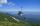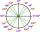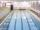Bevel

I have bevel in the ratio 1:6. What is the angle and how do I calculate it?

Result

x =  9.46 °

Solution:Leave us a comment of example and its solution (i.e. if it is still somewhat unclear...):Be the first to comment!To solve this verbal math problem are needed these knowledge from mathematics:

Need help calculate sum, simplify or multiply fractions? Try our fraction calculator. See also our right triangle calculator. Most natural application of trigonometry and trigonometric functions is a calculation of the triangles. Common and less common calculations of different types of triangles offers our triangle calculator. Word trigonometry comes from Greek and literally means triangle calculation.

Next similar examples:

1. High wallI have a wall 2m high. I need a 15 degree angle (upward) to second wall 4 meters away. How high must the second wall?Average climb of the road is given by ratio 1:15. By what angle road average climb?
3. StairwayWhat angle rising stairway if step height in 17 cm and width 27 cm?
4. ReflectorCircular reflector throws light cone with a vertex angle 49° and is on 33 m height tower. The axis of the light beam has with the axis of the tower angle 30°. What is the maximum length of the illuminated horizontal plane?On a straight stretch of road is marked 12 percent drop. What angle makes the direction of the road with the horizontal plane?
6. Map - climbOn the map of High Tatras in scale 1:11000 are cable car stations in the Tatranska Lomnica and in the Skalnate Pleso with distance 354.6 mm. Altitude of this stations are 949 m and 1760 m. What is average angle of climb of this cable car track?What is greater angle? -0.7 radians or -0.7π radians?
8. PyramidPyramid has a base a = 5cm and height in v = 8 cm. a) calculate angle between plane ABV and base plane b) calculate angle between opposite side edges.
9. Slope of the poolCalculate slope (rise:run) of the bottom of swimming pool long 10 m. Water depth at beginning of pool is 1.16 m (for children) and depth at end is 1.89 m (for swimmers). Slope express as percentage and as angle in degrees.
10. Theorem proveWe want to prove the sentence: If the natural number n is divisible by six, then n is divisible by three. From what assumption we started?
11. TreeHow tall is the tree that observed in the visual angle of 52°? If I stand 5 m from the tree and eyes are two meters above the ground.
12. LiftThe largest angle at which the lift rises is 16°31'. Give climb angle in permille.
13. CosineThe point (8, 6) is on the terminal side of angle θ. cos θ = ?
14. MapleMaple peak is visible from a distance 3 m from the trunk from a height of 1.8 m at angle 62°. Determine the height of the maple.
15. ClimbRoad has climbing 1:27. How big is a angle corresponds to this climbing?
16. Reference angleFind the reference angle of each angle:
17. PowersExpress the expression ? as the n-th power of the base 10.